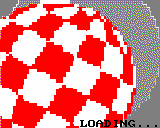# b8

## Boing Ball

### PreviewGitHub

### Code at a glance

``````REM Boing Ball - Entry program
REM Recreated of the classic Amiga demo.
REM Based on Toddl's work:
REM   http://lowres.inutilis.com/programs/?lccpost=YDxBl4UZxp.
REM
REM Press Ctrl+R to run.

REM Initializes the driver.

drv = driver()
print drv, ", detail type is: ", typeof(drv);

REM Constants.

' Colors.
RED = rgba(255, 0, 0)
WHITE = rgba(255, 255, 255)
' How many radians is the ball tilted.
ROTATE_ANGLE = 0.5

REM Resources.

REM Variables.

white_start = 1
ball_x = 5
parabel_x = 0
ball_dir = 1
parabel_dir = 9.25

REM Functions.

' Shows the background.
def background()
col rgba(225, 150, 225)
for y = 0 to 111 step 16
for x = 23 to 134 step 16
rect x, y, x + 17, y + 17
next
next
for x = 23 to 135 step 16
line x, 112, (x - 80) / 96 * 160 + 81, 127
next
line 15, 116, 144, 116
line 3, 121, 155, 121
line 0, 127, 159, 127
enddef

' Cycles the palette.
def cycle_palette()
white_start = white_start - ball_dir
if white_start > 13 then white_start = 2
if white_start < 2 then white_start = 13
for ci = 0 to 11
if ci < 6 then
pv = RED
else
pv = WHITE
endif
c = (ci + (white_start - 2)) mod 12 + 2
pset c, pv
next
enddef

' Draws one line of the ball.
def plot_line(x)
for y = 0 to PI step 0.008
c0 = (floor((x + PI) * 15 + 0.5)) mod 12 + 2
c1 = ((c0 - 2) + 6) mod 12 + 2
v = 0
if y * 2.5 mod 2 = 0 then
v = c0
else
v = c1
endif
xx = sin(y) * cos(x) * 30
yy = cos(y) * 30
s = sin(ROTATE_ANGLE)
c = cos(ROTATE_ANGLE)
posx = xx * c - yy * s
posy = xx * s + yy * c
sset the_ball, 1, floor(posx + 30.5), floor(posy + 30.5), v
next
enddef

' The main loop.
def update(delta)
' Shows the background.
background()
' Processes bouncing.
parabel_x = parabel_x + parabel_dir * delta
ball_y = parabel_x * parabel_x * 1.5
if ball_y >= 45 then
ball_y = 45
parabel_x = sqr(ball_y / 1.5)
parabel_dir = -parabel_dir
endif
' Moves the ball left and right.
if ball_x <= 0 then
ball_x = 0
ball_dir = -ball_dir
sfx 5,110,0.08
elseif ball_x >= 99 then
ball_x = 99
ball_dir = -ball_dir
sfx 5,110,0.08
endif
ball_x = ball_x + ball_dir * delta * 25
' Shows shadow of the ball.
clip 23, 0, 113, 128
circfill ball_x + 40, ball_y + 37, 30, rgba(20, 20, 20, 110)
clip
' Cycles palette and shows the ball.
cycle_palette()
spr the_ball, ball_x, ball_y + 18
if ball_y >= 45 then
sfx 5,80,0.1
endif
enddef

' Prefabs the chequered ball.
for i = 0 to 1